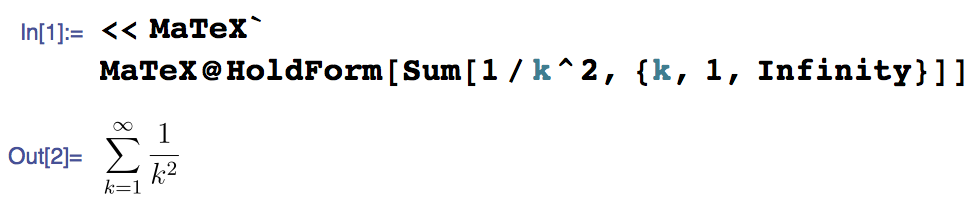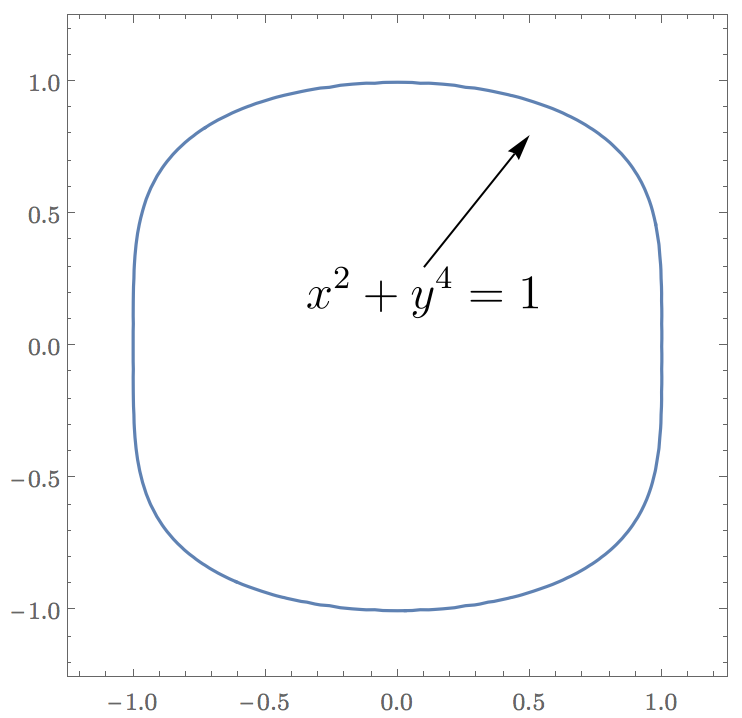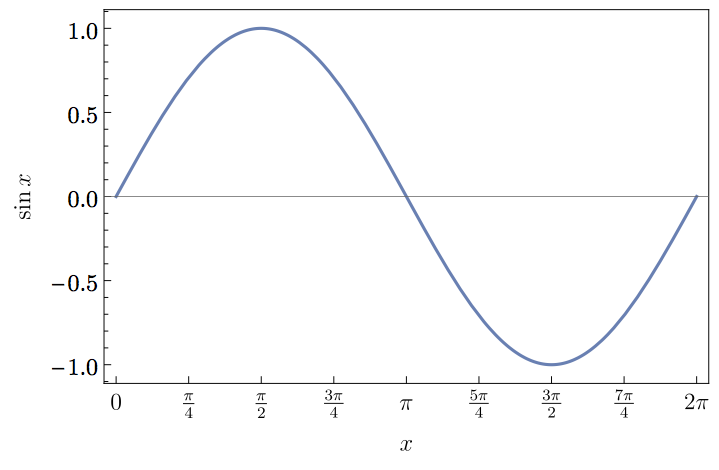# MaTeX:在Mathematica的图片中插入LaTeX代码的工具

669 篇文章 0 订阅

Mathematica图片中对laTeX的支持实在不敢恭维。连Geogebra都比Mathematica强。

ConfigureMaTeX[ "pdfLaTeX" ->  "X:\\Program Files\\CTEX\\MiKTeX\\miktex\\bin\\pdflatex.exe",
"Ghostscript" -> "X:\\Program Files\\gs\\gs9.18\\bin\\gswin32c.exe"]

https://github.com/szhorvat/MaTeX/releases

https://github.com/szhorvat/MaTeX/archive/v1.6.3.zip

# LaTeX typesetting in Mathematica

Thu 05 March 2015

In Notes.

tags: mathematica

Mathematica is an excellent and flexible visualization tool, and even supports displaying complex mathematical formulae. However, its typesetting quality is not on par with LaTeX. The visual style is not a good match for inclusion in LaTeX documents either. To improve the quality of my figures, I wrote a small Mathematica package that makes it easy to use LaTeX-generated labels: MaTeX.

Update: The post below has been updated for MaTeX version 1.1.0. The changelog is available on GitHub.

There are already several solutions for this problem, such as PSTricks or drawing axes and labels withPGFPlots. None of these solutions make it easy to use a Mathematica-centric workflow though. MaTeX makes generating LaTeX-typeset expressions as simple asI originally wrote these functions for my own needs, but seeing that others may be interested in them too, I wrapped them up into a package. Feel free to leave comments below and keep in mind that this it is still a work in progress.

## Installation

To install MaTeX, download the latest release and drop the MaTeX.m file in the directory opened bySystemOpen@FileNameJoin[{$UserBaseDirectory, "Applications"}]. You will also need: • A TeX system that includes pdflatex with the standalone and lmodern packages. Both TeX Live and MiKTeX should work. • Ghostscript 9.15 or later.(Ghostscript 9.20) On OS X, MacTeX 2015 includes a compatible version of Ghostscript. If you use an older TeX distribution on OS X, get a recent Ghostscript from Richard Koch’s page. When loading MaTeX for the first time, it will try to automatically detect TeX and Ghostscript. If this fails, it will display instructions on configuring the location of the pdflatex and gs executables using theConfigureMaTeX command. As an example, on my OS X system I needed to use the following configuration: ConfigureMaTeX["pdfLaTeX" -> "/Library/TeX/texbin/pdflatex", "Ghostscript" -> "/usr/local/bin/gs"] The existing configuration can always be queried using the command ConfigureMaTeX[]. Note: On Windows use the c-suffixed command line Ghostscript executable, i.e. gswin64c.exe instead ofgswin64.exe. If you prefer, you can use xelatex instead of pdflatex. Unlike plain pdfLaTeX, XeLaTeX can load any installed system font. MaTeX should now be ready to use. Test it using MaTeX["x^2"]. ## Usage examples First we need to load MaTeX: <<MaTeX My primary use for it is creating figure annotations, as below: texStyle = {FontFamily -> "Latin Modern Roman", FontSize -> 12}; ContourPlot[x^2 + y^4 == 1, {x, -1.2, 1.2}, {y, -1.2, 1.2}, BaseStyle -> texStyle, Epilog -> { Arrow[{{0.1, 0.3}, {0.5, 0.80}}], Inset[MaTeX["x^2+y^4=1", Magnification -> 2], {0.1, 0.3}, Scaled[{0.5, 1}]] }]Here I used the Latin Modern font for tick labels for visual consistency with the MaTeX/LaTeX output. We can also use MaTeX to generate beautifully typeset frame labels and frame ticks. Mathematica’s default frame and axes style is dark grey, while MateX outputs black. The BlackFrame style below makes the frames black too for consistency. Plot[Sin[x], {x, 0, 2 Pi}, Frame -> True, FrameStyle -> BlackFrame, FrameTicks -> {{Automatic, None}, {Table[{x, MaTeX[x, "DisplayStyle" -> False]}, {x, Pi/4 Range[0, 8]}], None}}, FrameLabel -> MaTeX /@ {"x", "\\sin x"}, BaseStyle -> texStyle]The MaTeX function can be used either with a string containing math-mode LaTeX code, or with an arbitrary Mathematica expression. It will automatically apply TeXForm to non-string expressions. When writing TeX code in Mathematica strings, remember to always escape backslashes. Thus \sum must be written as "\\sum". ## Advanced usage ### Options • "DisplayStyle". By default display style is used. Use "DisplayStyle" -> False for inline style. Inline formatting looks like k=11k2=π26 ∑k=1∞1k2=π26. Display style looks like k=11k2=π26 ∑k=1∞1k2=π26. • FontSize. Use it to set the font size. Note that LaTeX uses different glyph shapes for different font sizes to improve readability. Depending on the font used, only standard sizes may be available with this option. Use Magnification instead for proportional scaling. • "Preamble". This is a list of lines to be included in the LaTeX preamble. The default is{"\\usepackage{lmodern,exscale}", "\\usepackage{amsmath,amssymb}"}. The AMS packages are included by default because they may be required for compiling the output of Mathematica’s TeXForm, while lmodernprovides vector fonts and flexible font sizing. On most systems "\\usepackage{lmodern,exscale}" can be omitted. This option is most convenient to set permanently for the session, for example: SetOptions[MaTeX, "Preamble" -> {"\\usepackage{amsmath,amssymb}", "\\usepackage{color,txfonts}"}] MaTeX["\\color{red}\\sqrt{x}"] • Magnification. Set a scaling factor for MaTeX’s output. Magnification scales the result proportionally, unlike FontSize, which will use different glyph shapes for small text. ### Performance MaTeX needs to call pdflatex for every LATEX LATEX expression it generates. Each call might take as long as a second, which can be annoyingly slow when using MaTeX on many small expression, e.g. creating tick labels. As a partial remedy for this, MaTeX caches each result, so a second call with the same TeX code should be instantaneous. The maximum number of expressions to be cached can be controlled using ConfigureMaTeX["CacheSize" -> ...].ClearMaTeXCache[] will clear the cache. ## How does it work? MaTeX uses pdflatex and the standalone document class to create PDF files from TeX code. The height of thePDF files is ensured to be at least the line height by including a \strut. Mathematica can import PDF files, but it can only interpret simple ones correctly. To avoid problems, all font glyphs are converted to outline curves using Ghostscript before import. This capability is new in Ghostscript 9.15, hence the version requirement. ## Troubleshooting #### MaTeX no longer works on OS X 10.11 El Capitan If you use MacTeX on OS X El Capitan, the path to pdflatex or xelatex must be set as ConfigureMaTeX["pdfLaTeX" -> "/Library/TeX/texbin/pdflatex"] If you used the path /usr/texbin/pdflatex on OS X 10.10 or earlier, it will no longer work on 10.11. You must reconfigure MaTeX with the new path. Please see the explanation here. The path to Ghostscript is unchanged, /usr/local/bin/gs. #### “Invalid syntax in or before <!DOCTYPE html>” error This error indicates that MaTeX was not downloaded correctly. Please download the latest release of MaTeX.mfrom this page, and re-install it according to the instructions above. #### MaTeX::texerr on 32-bit Windows If you are using a 32-bit version of Mathematica on Windows, MaTeX may not work correctly and may show the following error every time the MaTeX function is used: MaTeX::texerr: Error while running LaTeX This is due to a bug in Mathematica for 32-bit Windows. Due to the rarity of this platform, I decided not to work around this problem. Please consider using the 64-bit version. If you must use the 32-bit version for some reason, contact me for partial workarounds. #### MaTeX still doesn’t work! Please restart Mathematica, then enter the following lines in a new notebook and evaluate them: Needs["MaTeX"] ConfigureMaTeX[] MaTeXDeveloper$Version

Save the notebook and email it to me along with a complete description of the problems you are seeing.03-04424
08-11368
05-31262
04-101176
09-02273
08-14146
08-085427
09-2032万+
12-162608
03-08166
07-044175点击重新获取扫码支付余额充值